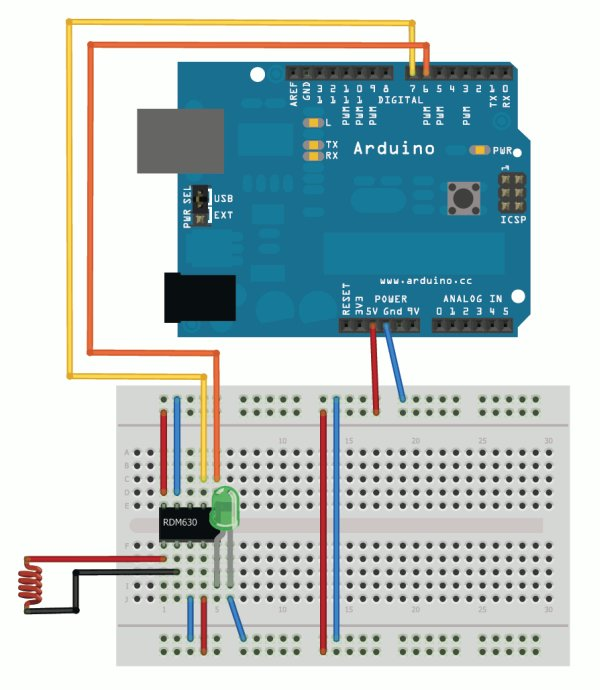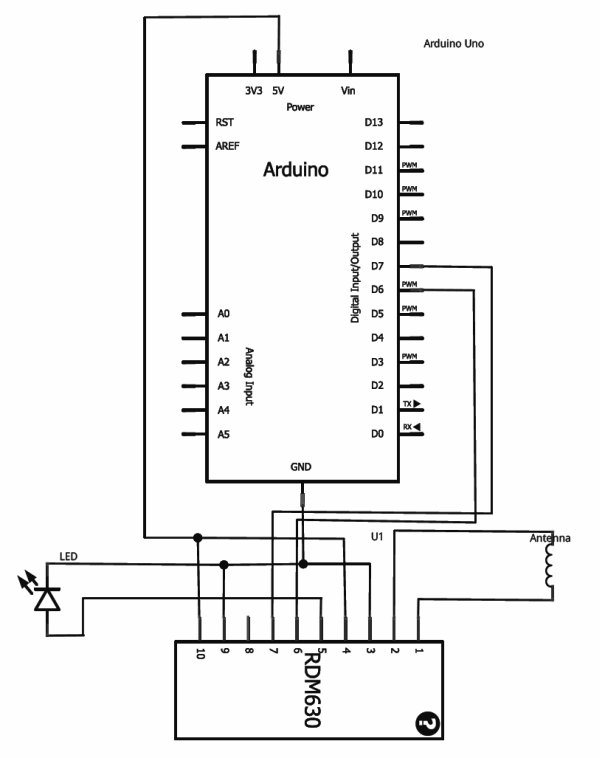# RFID with Arduino

If you ever wanted to integrate RFID functionality into your project, this small tutorial might help you accomplish that. I used the RDM630 module from seeedstudio in its UART version. It comes on a small board with presoldered connectors which fits perfectly on a breadboard. You only need to connect the antenna to the two pin socket. You also can connect a LED to indicate if the antenna picks up a signal. The signal output can be easily read by using theNewSoftSerial library and assigning the RX TX ports.The signal readings are transmitted in byte blocks. The first byte (02) is the start of text byte in HEX-ASCII format. Then following is the 10 byte data for the ID which has been recognized. After that a 2 byte checksum is transmitted. Followed by the last byte (03).
To translate the 10 byte HEX-ASCII data to the unique ID, you only have to map the bytes to the according ASCII character. For details have a look into the datasheet.

This is an example code which checks if the data was received correctly. It prints the ID and checks if the transmitted checksum is equal to the calculated checksum of the ID.

#include “NewSoftSerial.h”
#define stx 2
#define etx 3

NewSoftSerial mySerial(6, 7);
int counter;
byte data;
byte hexBlock1,hexBlock2,hexBlock3,hexBlock4,hexBlock5;
byte hexCalculatedChecksum,hexChecksum;

void setup() {
Serial.begin(9600);
mySerial.begin(9600);
}

void loop() {
if (mySerial.available() > 0) {
counter++;
if(counter > 13) {
//we read the whole message, so reset counter
counter = 0;
//check if start of text and end of text is correct
if(data == stx && data == etx) {
Serial.println(“Start of text and end of text correctly received.”);
Serial.print(“ID: “);
//show ID
for(int x = 1; x < 11; x++) {
Serial.print(data[x], BYTE);
}
Serial.println(“”);
Serial.print(“Checksum: “);
//show checksum
Serial.print(data, BYTE);
Serial.println(data, BYTE);

//Hex ID blocks. Two transmitted Bytes form one Hex ID block.
//Hex ID blocks:      6   2  |  E   3  |  0   8  |  6   C  |  E   D
//Transmitted Bytes: 36H 32H | 45H 33H | 30H 38H | 36H 43H | 45H 44H
hexBlock1 = AsciiCharToNum(data)*16 + AsciiCharToNum(data);
hexBlock2 = AsciiCharToNum(data)*16 + AsciiCharToNum(data);
hexBlock3 = AsciiCharToNum(data)*16 + AsciiCharToNum(data);
hexBlock4 = AsciiCharToNum(data)*16 + AsciiCharToNum(data);
hexBlock5 = AsciiCharToNum(data)*16 + AsciiCharToNum(data);

//Transmitted checksum.
hexChecksum = AsciiCharToNum(data)*16 + AsciiCharToNum(data);

//XOR algorithm to calculate checksum of ID blocks.
hexCalculatedChecksum = hexBlock1 ^ hexBlock2 ^ hexBlock3 ^ hexBlock4 ^ hexBlock5;
if ( hexCalculatedChecksum == hexChecksum )
{
Serial.println(“Calculated checksum matched transmitted checksum.”);
}
else {
Serial.println(“Calculated checksum didn’t match transmitted checksum. Corrupt data!”);
}
}
}
}
}

uint8_t AsciiCharToNum(byte data) {
//First substract 48 to convert the char representation
//of a number to an actual number.
data -= ‘0’;
//If it is greater than 9, we have a Hex character A-F.
//Substract 7 to get the numeral representation.
if (data > 9)
data -= 7;
return data;
}An example reading would look like this:

Start of text and end of text correctly received.
ID: 4200442C01
Checksum: 2B
Calculated checksum matched transmitted checksum.

Here is a demonstration of the RFID reader in action:

For more detail: RFID with Arduino